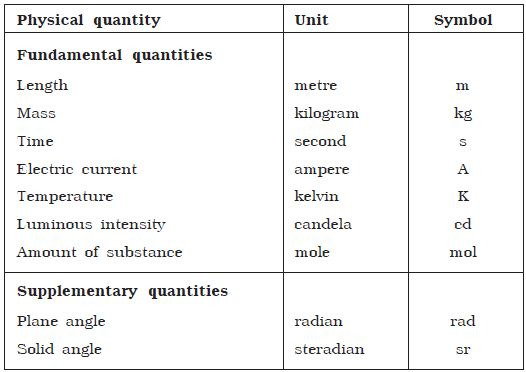Physics

# System International de Units

To measure a quantity, we always compare it with some reference standard. To say that a rope is 10 metres long is to say that it is 10 times as long as an object whose length is defined as 1 metre. Such a standard is called a unit of the quantity. Therefore, unit of a physical quantity is defined as the established standard used for comparison of the given physical quantity. The units in which the fundamental quantities are measured are called fundamental units and the units used to measure derived quantities are called derived units.

System International de Units (SI system of units)

In earlier days, many system of units were followed to measure physical quantities. The British system of foot-pound-second or fps system. the Gaussian system of centimetre – gram – second or cgs system. the metre-kilogram – second or the mks system were the three systems commonly followed. To bring uniformity, the General Conference on Weights and Measures in the year 1960, accepted the SI system of units. This system is essentially a modification over mks system and is, therefore rationalised m ksA (metre kilogram second ampere) system. This rationalisation was essential to obtain the units of all the physical quantities in physics.

In the SI system of units there are seven fundamental quantities and two supplementary quantities. They are presented below.